# Modulus on large numbers (more than" 2147483647").

How to calculate the rest of division on very large number in KNIME?
.
Indeed, inside the “Math Formula” node, the mod(x,y) or x%y function is limited to " 2147483647".
To avoid this limitation it’s possible to use the folowing process like (in VBA)

``````Function RestePar97(Nbre As String) As Integer
Dim i As Integer
RestePar97 = 0
For i = 0 To Len(Nbre) - 1
RestePar97 = (RestePar97 * 10 + CInt(Mid(Nbre, i + 1, 1))) Mod 97
Next i
End Function
``````

How can I transpose this function in KNIME?

Hi @PBJ ,

maybe i misunderstand your question - why not just use the long format in KNIME?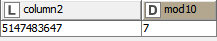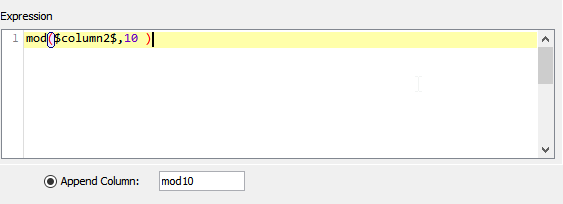Else you could use the java snippet node (or python nodes) to accomplish the same - but I think using long is easier to use (the limit here should be 9223372036854775807)

If you want mod for even larger calculations I would suggest to use java/python functions for large numbers e.g. bigint mod

Input: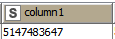Example:

Output: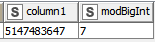Example workflow for both:
KNIME_project34.knwf (7.6 KB)

6 Likes

Hi @PBJ , the Math Formula is able to go beyond that. 2147483647 is basically the max value of a signed int (the range is -2,147,483,648 to 2,147,483,647). If you use type Long as @AnotherFraudUser pointed out, it will go beyond that number.

1 Like

By using a loop, I try to calculate the rest of a division on large integer numbers by using process like hand made.

The behavior of mod (on knime):

The initial numbers: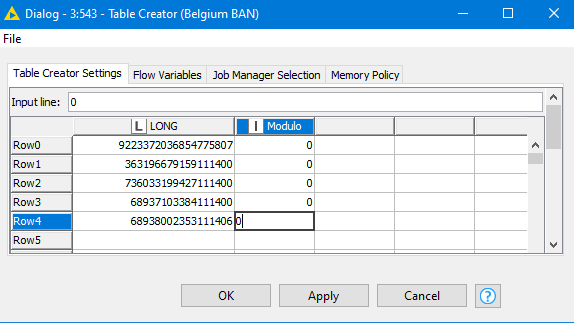The mod calculation:

The (wrong) results: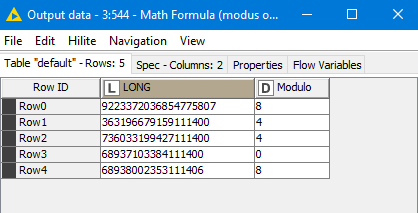I try to avoid Python (to avoid python installation) and have a self KNIME execution…Best regards.

2 Likes

Hi @PBJ,

it seems you are correct -it looks like there is a problem with incorrect casting thereAttached a java snippet with multiple ways to do the mod with the Java Snippet.
I think using these pre-build java function would be the correct way - or do you really want to build you self made function within knime?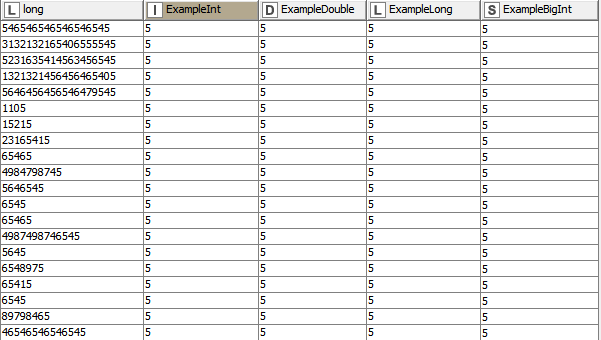KNIME_project34.knwf (7.0 KB)

Maybe some of the KNIME colleagues can give feedback (@ScottF) regadring the output of the math node (as well as column expression node)2 Likes

This issue seems to happen after a certain range/limit. I originally tested with some Long numbers, and they all passed, and I was about to reply that I did not have any issue on my side because of that, then I thought of testing with the numbers that @PBJ used, and unfortunately I got the same results too.

Some test results: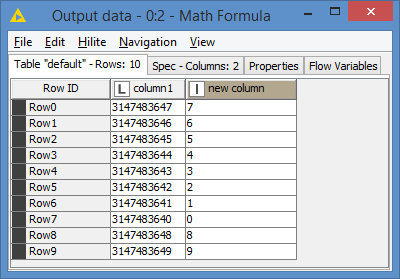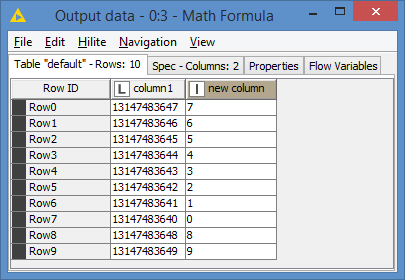Then I used the Math Formula (Multi Column) to go faster. At first, I did 4 columns in total (including the 2 above). Then I added @PBJ test data in column5. The first 4 columns produced the correct results, but for column5, it produced the same results as @PBJ:

I also processed column4 and column5 via Column Expression:

I’m guessing it’s the same `mod()` function in the Math Formula and the Column Expression.

But the issue might not be with the mod() function, but rather how these numbers are being recognized. Here’s an additional test that I did. I did column4 / 10 and also column5 / 10. Here are the results:

I’m not sure how these numbers are coming up.

Here’s my workflow if you want to play with these numbers: Math Formula mod issue.knwf (16.3 KB)

2 Likes

This topic was automatically closed 182 days after the last reply. New replies are no longer allowed.